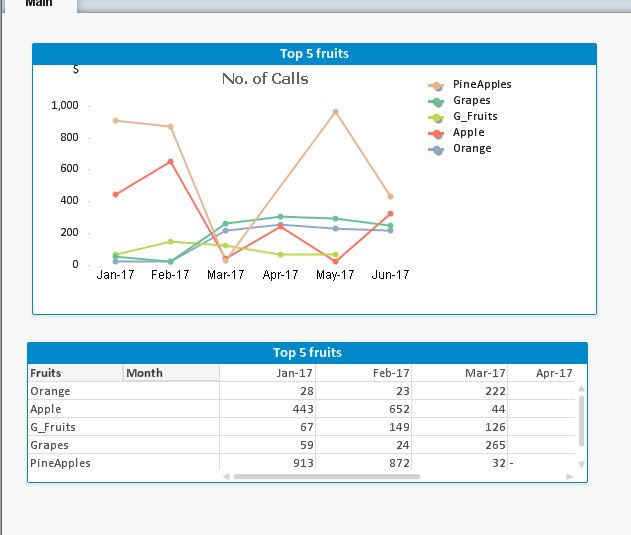# QlikView App Dev

Discussion Board for collaboration related to QlikView App Development.

Announcements
Now Live: Qlik Sense SaaS Simplified Authoring – Analytics Creation for Everyone: READ DETAILS
cancel
Showing results for
Did you mean:Contributor III

## Top 5 fruits selection not working in Pivot and Line chart

hi all,

Top 5 fruits selection not working in Pivot and Line chart. please find the attached samples.

TIA

1 Solution

Accepted SolutionsMaster II

Hi,

You can achieve in many ways,

1)  Calculated dimension

=aggr(only({<Fruits={'=rank(sum(Sales))<=5'}>} Fruits),Fruits)

or

=aggr(if(rank(sum(Sales))<=5,Fruits),Fruits)

2) Calculated measure using set analysis

SUM({<Fruits = {"=Rank(SUM(Sales),4)<=5"}>}Sales)

or  using aggr function

if(Aggr(Rank(Sum(Sales)),Fruits,Month)<=5,sum(Sales))

3) Using Dimension Limit (straight table)

Dimension Limits tab --> Fruits dimension, Select Show only Largest 5 Values

4) back end script method3 RepliesChampion III

What is your expected output should be ?Contributor III
Author

only top 5 sales should be visible in both the chartsMaster II

Hi,

You can achieve in many ways,

1)  Calculated dimension

=aggr(only({<Fruits={'=rank(sum(Sales))<=5'}>} Fruits),Fruits)

or

=aggr(if(rank(sum(Sales))<=5,Fruits),Fruits)

2) Calculated measure using set analysis

SUM({<Fruits = {"=Rank(SUM(Sales),4)<=5"}>}Sales)

or  using aggr function

if(Aggr(Rank(Sum(Sales)),Fruits,Month)<=5,sum(Sales))

3) Using Dimension Limit (straight table)

Dimension Limits tab --> Fruits dimension, Select Show only Largest 5 Values

4) back end script method